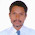## July 4, 2014

### SSC Maths Important Questions from New Syllabus in AP and Telangana

1 Mark Questions:

1. Find centroid of the triangle formed by A(-1, 2), B(0, 3), C(-2, 4) (P.S.)
2. How do you find the distance between two points on the line parallel to x-axis. Explain. (R & P)
3. If the slope of line segment joining P(-2, 3), Q(x, 6) is -1, then find x. (P.S.)
4. Find cubic polynomial with the zero values -7, 1, 2. (P.S.)
5. Can x+2, x+4 and x+9 be in A.P. Justify your answer. (R & P)

2 Marks Questions:

1. How many two digit numbers are divisible by 7 ? (Com)
2. Are sets of multiples of 3 and multiples of 2 disjoint sets. Justify your answer ? (R & P)
3. Find the ratio in which y-axis divides the line segments joining the points A(3, 2), B(-1, 2). (P.S.)
4. Find the area of quadrilateral formed by the points A(2, 1), B(4, 3), C(-1, 3), D(-3, 1) (P.S.)

4 Marks Questions:

1. The length and breadth of a rectangular metal sheet are in the ratio 7 : 5. Four 3cm I 3cm squares have been separated from the corners of that rectangle and it has been moulded into a cuboid of 96 cm3 of volume. Find the area of the rectangular metal sheet take in the beginning. (Connection)

2. A stone is thrown vertically upwards from a building of 96 ft height with a initial velocity of 116 ft/sec. If the acceleration due to gravity is 32 ft/sec2, then after how many seconds the stone will reach the ground. (Connection)

3. Rama has arranged 256 dots to draw a rangoli in the following ways. In how many rows has she arrange the dots. (P.S.)

4. The product of two consecutive multiples of 3 is 81. Form a quadratic equation and by using this information draw its graph. (Rep & V)

5. 5 women and 3 men having same capacity can complete a work in 6 days. And 3 men, 3 women of same capacity together complete the same work in 9 days, then in how many days a women or a man can complete the work. (P.S.)

### Related Posts

#### 1 comment:

1.gud evng sir. I want 10th class maths lesson plan model. could u please provide it. Abdul Rahiman